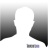Post# of 83010Re: IAMLEGEND post# 11996

Wednesday, 02/08/2012 7:04:52 PM

~IAMLEGEND LESSON OF THE DAY!!! ~FIBONACCI RETRACEMENT~Leonardo Pisano Bigollo (c. 1170 – c. 1250) also known as Leonardo of Pisa, Leonardo Pisano,Leonardo Fibonacci, or, most commonly, simply Fibonacci, was an Italian mathematician, considered by some "the most talented western mathematician of the Middle Ages.
He was one of the first people to introduce the Hindu-Arabic number system into Europe-the system we now use today- based of ten digits with its decimal point and a symbol for zero: 1 2 3 4 5 6 7 8 9. and 0

Fibonacci Numbers

The sequence, in which each number is the sum of the two preceding numbers is known as the Fibonacci series: 1, 1, 2, 3, 5, 8, 13, 21, 34, 55, 89, 144, 233, 377, 610, 987, 1597, 2584, 4181, ... (each number is the sum of the previous two).

Fibonacci trading simply involves measuring swing highs and lows and attempting to forecast price reversals and continuations.
Stocks will often pull back or retrace a percentage of the previous move before reversing. The three most common fibonacci retracement levels are 38.2%, 50%, and 61.8% of the primary trend and most basic stock charting applications will use these as standard levels. These fibonacci retracement levels act almost as magnets once the counter trend rally takes place. These are very common, however, there are a few other fibonacci levels that can provide resistance. These are the 75%, 78.6%, 87.5%, and 88.7% retracement levels. The common rule of thumb is that when the 50% retracement level is taken out, the four levels become magnets to attract price.Using Fibonacci retracements in your trading will not make you a professional swing trader, day trader or investor overnight. But used in conjunction with other technical analysis tools such as stochastics, RSI, MACD, moving averages, candlestick patterns, etc., they can be a very valuable addition to any traders tool box. Here is an example using a graphic explaining the retracement pattern:This picture shows a graphical representation of the reversal points for stocks in an uptrend. The pattern is reversed for stocks that are in down trends.

After a stock makes a move to the upside (A), it can then retrace a part of that move (B), before moving on again in the desired direction (C). These retracements or pullbacks are what you as a swing trader want to watch for when initiating long or short positions.

Once the stock begins to pull back (retrace), then you can plot these retracement levels on a chart to look for signs of a reversal. You do not automatically buy the stock just because it is at a common retracement level! Wait, and look for candlestick patterns to develop at the 38.2% area. If you do not see any signs of a reversal, then it may go down to the 50% area. Look for a reversal there. You do not know if or when the stock will reverse at a Fibonacci level! You just mark these areas on a chart and wait for signal to go long or short.How To Draw A Fib GridDraw the fib grid from the swing point high and the swing point low of a swing. Your charting software should come with this feature. It is a standard option on most charting packages. If not, you can calculate it manually by using this formula:

1.Calculate the range from the swing point high to the swing point low.
2. Multiply the range times a Fibonacci ratio: 38.2% (0.382), 50% (0.500), and 61.8% (0.618).

3.Subtract that number from the swing point high. That will give you your Fibonacci levels.
HS pulled back into the TAZ and then formed a bullish engulfing candle right at the 50% level

VIDEO-FIBONACCI RETRACEMENT

!

ExamplesConclusions

Fibonacci retracements are often used to identify the end of a correction or a counter-trend bounce. Corrections and counter-trend bounces often retrace a portion of the prior move. While short 23.6% retracements do occur, the 38.2-61.8% covers the more possibilities (with 50% in the middle). This zone may seem big, but it is just a reversal alert zone. Other technical signals are needed to confirm a reversal. Reversals can be confirmed with candlesticks, momentum indicators, volume or chart patterns. In fact, the more confirming factors the more robust the signal.* 20% Profit Rule